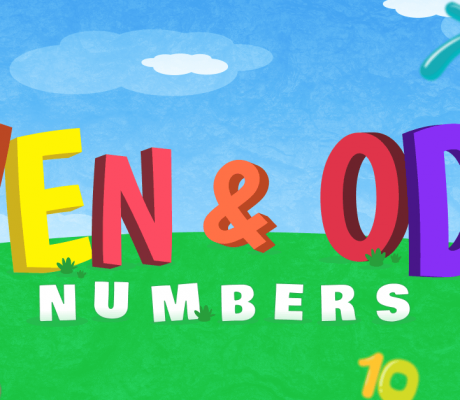### Even and Odd Numbers

What are Even and Odd Numbers? In mathematics, even numbers are integers divisible by 2, that is, there is no remainder when the integer is divided by two. On the other hand, odd numbers are integers, when divided by 2, will have a remainder of 1. Odd numbers are always in between even numbers and […]